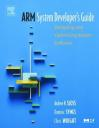I have worked on several platforms based on ARM cores: ARM7, ARM9 and XScale. ARM architecture has been present in more than 2 billion embedded products over the last 10 years, ranging from cell phones to automotive braking systems. I think ARM architecture is great for embedded computers and I needed to learn it so that I could get its best.The best book I found on this is ‘ARM System Developer’s Guide’ by Andrew Sloss (ARM Inc.), Dominic Symes (ARM Ltd.) and Chris Wright (Ultimodule Inc.). It covers all the ARM cores, XScale processors, demonstrates how to implement DSP algorithms, describes cache technologies that surround the ARM cores, as well as efficient memory management techniques.

Among the tasks I’ve had to deal with I’d point out Artificial Vision and Vocoders implementation for being computationally expensive.

In vocoders implementation and optimization case, I had to face some large of Digital Signal Processing issues which leaded me to write assembly code in order to get the best performance. Also I had to focus in the cache usage because it can speed up the execution time amazingly. Not to mention avoiding pipeline stalls and efficient use of the registers. The mentioned book covers all these topics both with theory and practical examples. There’s a dedicated chapter about DSP which even includes source code ready to use.

Even if you don’t need to write assembly code you can learn how to write efficient C code for ARM. I will show a simple example which can improve ‘intensive loop’ codes:

```int checksum(int *data)
{
unsigned int i;
int sum=0;
for(i=0; i<64; i++)
{
sum+=(*data++);
}
}```

This compiles to:

```	mov	r2,r0		; r2=data
mov	r0,#0		; sum=0
mov	r1,#0		; i=0
checksum_loop
ldr	r3,[r2],#4	; r3 = *(data++)
cmp	r1,#0x40	; compare i,64
bcc	checksum_loop	; if(i<64) loop
mov	pc,lr		; return sum```

The above code is not efficient, we can avoid the 3 steps loop: ADD 1 to i, comparison and the conditional branch instruction.

```int checksum_eff(int *data)
{
unsigned int i;
int sum=0;
for(i=64; i!=0; i----)
{
sum += *(data++);
}
return sum;
}```

As you can see, we just rewrote the loop to be descendent rathern than the previous incrementing loop. Let’s have a look at the compiled code:

```	mov	r2,r0			; r2=data
mov	r0,#0			; sum=0
mov	r1,#0x40		; i=64
checksum_2_loop
ldr	r3,[r2],#4		; r3=*(data++)
subs	r1,r1,#1		; i-- and set flags
bne	checksum_2_loop		; if (i!=0) loop
mov	pc,lr			; return sum```

As you can see, the loop work is done entirely by the subs and bne instructions. The comparison with zero is free since the result is stored in the condition flags. Thus we can see that it’s more efficient to make decrementing loops in ARM than incrementing ones.

Let’s have a look at the way one could have written the same function in C without taking efficiency into account:

```int checksum(int *cata)
{
char *i;
int sum=0;
for(i=0; i<64;i++)
{
sum += data[i];
}
}```

And the compiler output:

```	mov	r2,r0			;r2=data
mov	r0,#0			;sum=0
mov	r1,#0			;i=0
checksum_loop
ldr	r3,[r2,r1,lsl #2]	;r3=data[i]